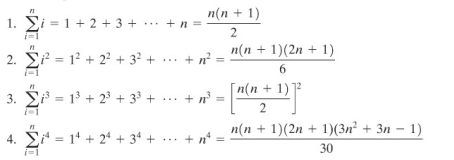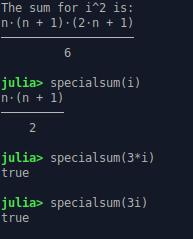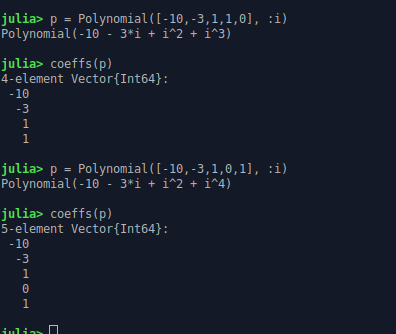# Help Me to Correct this Function for Special Sum with SymPy and CalculusWithJulia

Hi all,

I am trying to create a function and conditional loop inside,

I was creating this code:

``````using SymPy, CalculusWithJulia
@vars k n

function specialsum(k)
if k = i
k = (n*(n+1))/2
elseif k = i^2
k = (n*(n+1)*(2n+1))/6
elseif k = i^3
k = ((n(n+1))/2)^2
elseif k = i^4
k = (n*(n+1)*(2n+1)*(3n^2 + 3n - 1))/30
else
k = k
end
return
end

x = 7i

println("The sum for \$x is:")

k = specialsum(x)
``````

Error occured:
WARNING: Method definition (::Type{Base.Order.Lt{T} where T})(Function, Real) in module ImplicitEquations at /home/browni/.julia/packages/ImplicitEquations/YhI8o/src/predicates.jl:55 overwritten in module CalculusWithJulia at /home/browni/.julia/packages/CalculusWithJulia/Z8IBs/src/plot-utils.jl:16.
** ** incremental compilation may be fatally broken for this module ****

Stacktrace:
**  top-level scope**
** @ ~/LasthrimProjection/plot.jl:5**
**  include(fname::String)**
** @ Base.MainInclude ./client.jl:451**
**  top-level scope**
** @ REPL:1**
in expression starting at /home/browni/LasthrimProjection/plot.jl:5

The idea is when I put `x=7i` or `x=\$i^2\$` then it should print the special sum formula in `n` terms.

Like this screenshotThus,

i = n(n+1)/2 and so on

This (and similar) lines have a couple of issues.

• Checking for equality is done with `==`. On the other hand `=` is for assignment only.
• `i` is not defined anywhere, so neither we nor the compiler know what `i` is supposed to be.

It is not very clear what you are trying to do. I am not sure what you mean by “when I put x=7i it should print the special sum formula in n terms like this screenshot”. In the screen shot `i` is a “muted” variable which just describes what you are summing over, so it is confusing when you then say `i=n(n+1)/2` as `i` is not supposed to have a value outside of the loop according to your screenshot.

I would suggest putting your error messages also in code blocks, so that they are easier to read.

You mentioned you are trying to make a conditional loop. Are you on purpose using only `if-else` statements without a typical loop construct (like `for` or `while`)?

1 Like

Hi @Krastanov ,

thanks for the information,

I understand that `k = ..` that I use is wrong, I should have used `k ==...`

The point is when I put 7i, the answer should be 7*(n*(n+1))/2
the sum symbol (sigma from i=1 to n) will not be shown.

This is almost working I think but does not show the result that I wanted `7*(n*(n+1))/2`

``````using Symbolics, SymPy, CalculusWithJulia
@vars k n i

function specialsum(k)
if k == i
k = (n*(n+1))/2
elseif k == i^2
k = (n*(n+1)*(2n+1))/6
elseif k == i^3
k = ((n(n+1))/2)^2
elseif k == i^4
k = (n*(n+1)*(2n+1)*(3n^2 + 3n - 1))/30
else
k == k
end
return
end

x = 7i

println("The sum for \$x is:")

specialsum(x)
``````

It’s working for `i, i^2, i^3`, but not if I put `2i, 3i, ...` you can check my code

``````using Symbolics, SymPy, CalculusWithJulia
@vars k n i

function specialsum(k)
if k == i
return k = (n*(n+1))/2
elseif k == i^2
return k = (n*(n+1)*(2n+1))/6
elseif k == i^3
return k = ((n(n+1))/2)^2
elseif k == i^4
return k = (n*(n+1)*(2n+1)*(3n^2 + 3n - 1))/30
else
return k == k
end
return
end

x = i

println("The sum for \$x is:")

specialsum(x)
``````1. How can we tackle the scenario of `2i + i^2` for example

2. Is it better to use `for` or `while` than `if else`?

Could you try to talk through (1) what you want the answer to be when your input is `2i` (you have not really explained what it is supposed to do in such a situation) and (2) why are you trying to do what you are trying to do. I suspect the issue is a bit more fundamental than just syntax or coding style: why should the answer be `7*n*(n+1)/2` if the input is `7i`? That does not yet make sense to me

Also a few minor things:

• just use `return n*(n+1)/2`, not `return k = n*(n+1)/2`
• what is the point of `return k==k`
• the very last return does not seem to ever get reached
• you do not need to do `@vars k`, as you never use a symbolic object stored in the label `k`, rather `k` is used as a label only as an input variable for the function definition `specialsum`. It would really help you to learn the “variable scoping rules” that julia employs and to learn the difference between “julia symbol” / “julia variable” / “symbolic object from SymPy that happens to be stored in a julia variable”
• Symbolics and SymPy do not work together: they are two different implementations of the same type of tools so importing them at the same time would cause you only confusion - just pick one of them

It would help if you try to define in English what the input to the function `specialsum` is supposed to be. It seems you are conflating two different definitions:

• the input is supposed to be the “shape” of the term in the sum. I.e. if the input is `sin(i)` then the sum should be `sum([sin(i) for i in 1:n])`, but done symbolically for an arbitrary `n`
• this is probably wrong impression of mine, but it is the source of my confusion: the input is supposed to be the number of terms in the sum, i.e. something more like `n`, I am saying this because of your `7i` comment, which does not make much sense to me. Maybe you meant “for input of 7i” the output should be `7n * (7n+1) / 2`" (notice the second factor of 7 you missed).

Under the assumption that your goal is to write the first function, I would suggest doing it in the following way:

1. write a function `linear_sum(n) = n*(n+1)/2` and the function `quadratic_sum(n) = n*(n+1)*(2n+1)/6` and so on.
2. write a function that:
• takes a symbolic expression
• checks that the expression is a polynomial of order smaller or equal to 4 (raise an error if that is not the case)
• extract the monomials of each order, and for the linear order compute `weight*linear_sum(n)`, for the second order compute `weight*quadratic_sum(n)`, etc
• e.g. if the first order term is `2*i` compute `2*linear_sum(n)`
• e.g. if the second order term is `5*i^2` compute `5*quadratic_sum(n)`
• sum these terms and return them
• decide on some rules for how `i` and `n` are defined; maybe for a first implementation it makes sense for them to be just some global symbolic objects

I guess this means that the only thing you need to learn is how to decompose symbolic expressions into polynomial terms. It is not well documented but it seems to be described in here implement `coeff` by t-bltg · Pull Request #677 · JuliaSymbolics/Symbolics.jl · GitHub

Hi @Krastanov ,

about your writings, it helps me a lot, but this link to obtain the coefficients does not work for me:

`````` UndefVarError: coeff not defined

``````

But, then I try Polynomials.jl to gain the degree. So instead of making input in function, I make input in term of Polynomials, that the coefficients can be extracted in vector. But the problem is if I make the matrix a as `a = [n 2n 3n 4n n]`, then `a*(coeffs(p))` can be easily obtained, but the matrix a is not only n with degree 1.

``````using Symbolics, Polynomials
@variables n i

a = [1 (n*(n+1))/2 (n*(n+1)*(2n+1))/6 ((n(n+1))/2)^2 (n*(n+1)*(2n+1)*(3n^2 + 3n - 1))/30]

# 1 + 2i -> Polynomial([1,2,0,0], :i)
# 3i^2 + i^4 -> Polynomial([0,0,3,0,1], :i)

p = Polynomial([0,0,3,0,1], :i)

# coeffs(p) -> returns the entire coefficient vector
# Polynomials.degree(p) -> returns the polynomial degree, length is 1 plus the degree
println("The special sum for \$p is:")
a*(coeffs(p))
``````

thus the code above suppose to be working if I can manage to solve this error:

``````Sym n is not callable. Use @syms n(var1, var2,...) to create it as a callable.
Stacktrace:
 error(s::String)
@ Base ./error.jl:33
 (::SymbolicUtils.Sym{Real, Base.ImmutableDict{DataType, Any}})(args::SymbolicUtils.Add{Real, Int64, Dict{Any, Number}, Nothing})
@ SymbolicUtils ~/.julia/packages/SymbolicUtils/qulQp/src/types.jl:172
 (::Num)(args::Num)
@ Symbolics ~/.julia/packages/Symbolics/HDE84/src/num.jl:17
 top-level scope
@ REPL:1

``````

P.S. you are right `7i` is supposed to be `7n * (7n+7) / 2`, I have lots of typos.

Polynomials, Symbolics, (and SymPy) are three unrelated libraries that do not work together by default. I would suggest sticking to only one of them for the moment.

I still use Symbolics and Polynomials, it almost finish but still problematic, if I only put the Polynomials till degree 3, then it should have been appended for degree 4 and 5 to have 0 coefficient.

This code is working:

``````using Symbolics, Polynomials
@variables n i

#a = [1 (n^2+n)/2 n*(n+1)*(2n+1)/6 (1/4)*(n*(n+1))^2 (n*(n+1)*(2n+1)*(3n^2 + 3n - 1))/30]
a = [1 n*(n+1)/2 n*(n+1)*(2n+1)/6 (1/4)*(n*(n+1))^2 (n*(n+1)*(2n+1)*(3n^2 + 3n - 1))/30]

# 1 + 2i -> Polynomial([1,2,0,0], :i)
# 3i^2 + i^4 -> Polynomial([0,0,3,0,1], :i)
# i^2 - 3i - 10 -> Polynomial([-10,-3,1,0,0], :i)

p = Polynomial([-10,-3,1,1,1], :i)

# coeffs(p) -> returns the entire coefficient vector
# Polynomials.degree(p) -> returns the polynomial degree, length is 1 plus the degree
println("The special sum for \$p:")
println("is:")
a*(coeffs(p))

#collect(Polynomials.monomials(p))

``````

But, if I change the polynomial into:

`p = Polynomial([-10,-3,1,0,0], :i)`

then `coeffs(p)` become only of degree 3, but I want it to be still the same 5-element vector like this `[-10; -3; -1; 0; 0]`